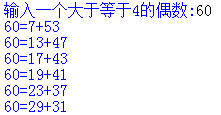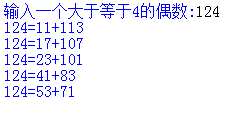2021-05-21 11:03

# python利用该函数验证哥德巴赫猜想

实例1输入一个大于等于4的偶数60

60=7+53

60=13+47

60=17+43

60=19+41

60=23+37

60=29+31• 点赞
• 写回答
• 关注问题
• 收藏
• 邀请回答

#### 2条回答默认 最新

•已采纳

源程序如下：

``````#判断是否是素数
def isPrime(n):
for i in range(2,n-1):
if n % i == 0:
return False
else:
return True

n = int(input("请输入一个大于4的偶数:"))
for i in range(2,n//2):
if (isPrime(i) and isPrime(n-i)):
print("%d=%d+%d\n"%(n,i,n-i))``````

运行结果：

``````请输入一个大于4的偶数:60
60=7+53

60=13+47

60=17+43

60=19+41

60=23+37

60=29+31``````
点赞 打赏 评论
•小P聊技术 2021-05-21 11:17
``````import math
def isPrime(n):
if n==1:
return False
elif n==2:
return True
else:
for i in range(2,int(math.sqrt(n))+1):
if n%i==0:
return False
return True

def check_num():
k = 0
for i in range(4,2000,2):
for j in range(1,i):
n2 = i -j
if isPrime(n2) and isPrime(j) and j<=n2:
print(j,"+",n2,"=", i,end="   ")
k+=1
if k%6==0:
print("\n")

check_num()``````
点赞 打赏 评论